Estimating Node Density for Redundant Sensors in Wireless Sensor Network | OMICS International
International Journal of Sensor Networks and Data Communications

# Estimating Node Density for Redundant Sensors in Wireless Sensor Network

Dhruv Sharma1*, Kayiram Kavitha2 and R Gururaj3

1Software Engineer, PayPal, Chennai, India

2Researcher, Pune, India

*Corresponding Author:
Dhruv Sharma
Software Engineer
Paypal, L404 Lancor Appt
Central Park South, Sholinganallur
Tel: +919666852383
E-mail: [email protected]

Received date: October 12, 2015; Accepted date: November 03, 2015; Published date: November 06, 2015

Citation: Sharma D, Kavitha K, Gururaj R (2015) Estimating Node Density for Redundant Sensors in Wireless Sensor Network. Sensor Netw Data Commun 4:127. doi:10.4172/2090-4886.1000127

Copyright: © 2015 Sharma D, et al. This is an open-access article distributed under the terms of the Creative Commons Attribution License, which permits unrestricted use, distribution, and reproduction in any medium, provided the original author and source are credited.

Visit for more related articles at International Journal of Sensor Networks and Data Communications

#### Abstract

The lifetime of a sensor network depends on the judicious utilization of the resource-constrained nodes. Practices like data aggregation, sleep scheduling play a major role in conserving the node’s energy. But in most cases, we observe a disparity in energy consumption rates among different sensors. This disparity results from higher utilization of a small set of deployed sensors in the field leaving these sensors drained out of power. To overcome this problem, it is often required to deploy redundant sensors to act as replacements for a faulty node. Secondly, the sensor network technology, being an application oriented technology, experiences variance in network parameters from application to application because of the various dynamics in nature. It is not often viable to go for a theoretically determined sensor distribution technique. Thus, it is often required to place sensors by studying the geographical constraints. These have proven to be highly valuable in designing energy efficient routing schemes anThe lifetime of a sensor network depends on the judicious utilization of the resource-constrained nodes. Practices like data aggregation, sleep scheduling play a major role in conserving the node’s energy. But in most cases, we observe a disparity in energy consumption rates among different sensors. This disparity results from higher utilization of a small set of deployed sensors in the field leaving these sensors drained out of power. To overcome this problem, it is often required to deploy redundant sensors to act as replacements for a faulty node. Secondly, the sensor network technology, being an application oriented technology, experiences variance in network parameters from application to application because of the various dynamics in nature. It is not often viable to go for a theoretically determined sensor distribution technique. Thus, it is often required to place sensors by studying the geographical constraints. These have proven to be highly valuable in designing energy efficient routing schemes and network topologies for sensor networks. In this paper we propose a scheme to decide how the distribution of available redundant sensor nodes should take place around sensor nodes. The scheme gives the flexibility to determine sensor positions based on application and geographical constraints. We propose to use the probability estimates of the utilization of a sensor in a given deployment to achieve desired network lifetimes. We also show how in some cases we can leverage the relative position from source(s) and sink be used for the same.d network topologies for sensor networks. In this paper we propose a scheme to decide how the distribution of available redundant sensor nodes should take place around sensor nodes. The scheme gives the flexibility to determine sensor positions based on application and geographical constraints. We propose to use the probability estimates of the utilization of a sensor in a given deployment to achieve desired network lifetimes. We also show how in some cases we can leverage the relative position from source(s) and sink be used for the same.

#### Keywords

Wireless sensor network; Deployment of nodes; Energyefficiency; Probabilistic analysis

#### Introduction

A Wireless Sensor Network (WSN) is a collaboration of a large number of sensor nodes. The sensor node operates on small batteries with limited lifetime. Some sensors, acting as source nodes, transmit their sensed data to a sink node via multi-hop communication. Often nodes nearer to the sink expend more energy than the nodes farther from the sink. Therefore, it is highly desirable to have an efficient sensor node distribution. The sensor nodes are often deployed in remote geographical locations or hazardous environment, where the replacement of batteries is very difficult and expensive. Therefore the prime consideration in WSN is to prolong the battery lifetime by efficient utilization. It is observed that the energy spent in routing data is about 80% of the total energy in the network while the remaining energy is used in sensing and other operations. Hence, various schemes were proposed in the past for energy efficient data routing, data aggregation, query processing etc. in a WSN.

Network topology is also one of the fundamental issues in wireless sensor networks (WSNs) that affects not only routing but also energy efficiency in a WSN. An efficient topology can reduce the number of hops in the network aiding to energy efficiency. The sensors are spread across the region of interest for satisfactory coverage. A deployment strategy determines the number of sensor nodes required in the Region of Interest (ROI). The deployment strategy impacts the routing schemes. At present, the focus is on developing power-efficient topologies  and routing schemes  that ensure not only the neighbors are at appropriate distances but the next hop for transmission is chosen based on the energy availability of neighboring nodes.

In WSNs, network deployments can be broadly categorized into: random and deterministic deployments. The geographic locations like volcano, seismic zone etc., are physically inaccessible. Here, the sensor nodes are deployed by means of a helicopter or any other means and termed as random deployment. As the sensor node location cannot be determined, the deployment strategy is termed as random deployment. This often leads to randomly distributed node densities in various portions of the network.

In contrast, deterministic deployments are preferred in scenarios when the deployment area is physically accessible. Deterministic techniques focus on coverage, network longevity, improving connectivity and improving data reliability. Not just that, deterministic schemes have more control over placement of the nodes and also provide a lower bound on the number of nodes needed to cover the area which proves helpful in achieving pre-determined performance.

A considerable research has been done in formulating algorithms  to determine the optimal node locations to achieve maximum coverage, connectivity and network lifetime. But, it does not consider application specific factors like inaccessible terrains; environmental obstructions etc. Therefore, deterministic deployments after careful sampling of environment specific factors are becoming increasingly popular. The City Sense  network for urban monitoring, the Soil Monitoring  etc., are some of the cases where sensors are placed by monitoring physical conditions of the application. Significant work has been done in estimating the node density and number of redundant nodes required in a WSN application. The density of redundant nodes is estimated based on the distance of a node from the sink while dealing with connectivity and coverage.

In general, the sensors closer to the sink tend to consume more energy than those farther from the sink. The reason for this disparity is that the sensors closer to the sink are sending data, sensed by them, to the sink as well as routing the transmissions originating at the nodes further from the sink. Due to the higher rates of energy consumption, the sensors closer to the sink die early leaving the sink disconnected with a portion of the network. This disparity has a serious impact on network lifetime and connectivity. The work in  proposed that the sensor density should increase from source to sink. However, it was assumed that each node is equally likely to serve as the source for forwarding its data to the sink. This scenario is not true in all cases. In some cases it is possible that only outermost nodes or nodes unevenly distanced from the sink serve as sources. In such a case, the observation that the node density should increase from source to sink would not hold true and we have to look for an alternative approach to determine the critically affected regions.

Deploying unequal number of redundant sensors around each sensor is also an effective measure to resolve the discrepancies in energy consumption. A node whose area of coverage overlaps with another node is often regarded as a redundant node. The idea is to first cover the ROI with a minimal number of sensor nodes using any distribution scheme and topology, as the same is subject to application conditions, and then deploy the remaining nodes as redundant nodes around each sensor. In this paper we propose a simple concept exclaiming that the number of redundant nodes around a particular sensor should be proportional to the estimate of the energy consumption by the sensor. We first prove that in certain scenarios it’s not always the best solution to increase density of redundant nodes from source to sink (Section 5). Then, for n-neighbor topologies, we show the hop-distance from the sink and source can be used to derive an estimate for the number of redundant nodes.

The rest of the paper is organized as follows. The literature survey is discussed in 4. In 5 we show, by comparing a geometrically increasing and uniform distribution model, that an increasing distribution model cannot prove to be the best solution for all scenarios. In Section 6, 7 and 8 we present the proposed scheme with description, simulation and experimental results. Finally, we conclude the work in Section 9.

#### Literature Survey

In this section we discuss the various approaches for achieving desired network lifetime challenged by disparity in energy consumptions in different regions of the network. There are a lot of deployment schemes [6-9] to determine node densities in different regions of the network suggested for the achieving elevated network lifetime in a scenario where sensors have different rates of energy consumptions. Often deploying redundant sensors around each sensor based on its distance from a sink  is considered to be an effective measure to solve the problem. The scheme proposed in  is most related to our work as we determine an estimate number for these redundant sensors to be deployed in different regions of the network based on its estimated utilization in the course of the network’s lifetime.

In certain scenarios, it is observed that the nodes closer to the sink tend to experience more traffic than other nodes. As a result, their energy consumption rates tend to be higher than those nodes that are distant from the sink. The nodes closer to the sink tend to die early leaving a hole near the sink and therefore, disconnecting the sink from some nodes in the network. This phenomenon is common in WSNs where the sensor nodes are homogeneous and report events generated at a constant rate to the sink and is known as the energy-hole problem [10,11].

One of the solutions to the energy-hole problem is proposed in . In this scheme, the first task is to divide the entire Region of Interest (ROI) into concentric coronas or rings around the sink. Then, one of the sensors in each ring is chosen to forward data from outmost rings to the sink at equal intervals. A message transmitted from the outermost ring (say) Ci is forwarded by sensor nodes in Ci−1, Ci−2 to the sink (centre of the circle) as shown in Figure 1. With a goal to achieve an equal energy dissipation rate in all rings except the outermost one, the authors proposed that the number of nodes should increase geometrically from the outermost ring towards the sink. This is also observed in the deployment scheme proposed in  where nearly balanced energy depletion in the network is achievable by deploying a geometrically increasing node density from the outer coronas to the inner ones. The work in  proposed a technique to estimate the number of nodes to be deployed to achieve a predetermined lifetime. The ROI is divided into equal sized strips. Here the density of sensors deployed increases as the distance between a strip and the sink decreases. Hence the distribution is effective.

Figure 1: Deployment of concentric rings with (a) Uniform sensor distribution, (b) Non-uniform sensor distribution.

In  the author proposes a sensor deployment strategy for tree based data forwarding observing the sleep-scheduling scheme used for sensor nodes. Here, the root of the tree acts as a source, forwarding data to a sink, which may be one of the leaf nodes. It estimates number of nodes in the rooted sub-tree of an intermediate node in the data forwarding tree. Based on the estimation, the number of redundant nodes required to be placed is calculated. The model assumes each region is w-covered by sensor nodes, out of which one node is in active state and others are redundant. An intermediate node in the data forwarding tree should have at least z × W number of redundant nodes where z increases from the source towards the sink.

We propose to increase the network longevity by estimating only the number of redundant nodes to be deployed around each sensor given an upper limit on the total number of sensor nodes that can be deployed in the network. However, the fundamental assumption in the above works is that all sensors are likely to act as source which leads to the observation that the number of redundant nodes or the node density should increase as we move from source/root towards sink. In Section 5 we show that the above observation (z, the number of redundant nodes, should increase from root to sink) is not true in some models.

#### Limitations in Existing Approach

As discussed in Section 4, a geometrically increasing node distribution is favorable solution for the energy-hole problem. The underlying assumption in these schemes was that each sensor transmits its data to a central static sink. This model is known as a Single Static sink Centre Placement (SSCP) deployment model. Secondly, in applications such as volcano monitoring , where the sensor nodes are placed only in safe areas near gas plumes, it cannot be assumed that each sensor would act as a source sensor at all times. There may be nodes that act only as relays to constitute a multi-hop communication between the source sensors and the sink. Thus, it is important to study whether the same observation (a geometrically increasing node distribution) holds true for such scenarios. In this section we try to prove that “an increasing distribution model cannot prove to be the best solution for all scenarios”.

In this section, we assume two popular node distribution models, mainly an increasing node distribution for redundant nodes from source to sink and a uniform distribution of redundant nodes and run simulations to understand how the network lifetime varies for both of them under different scenarios. The simulation environment is explained in Section 8. Further, we simulate our experiments over different scenarios based on the following factors:

1) The sensors send data to the sink acting as source nodes, i.e., the set of sensors acting as source during the simulation.

a. Each node acts as a source, forwarding data to the sink at equal intervals,

b. Only the end nodes or the nodes in the outermost rings act as the source with each one sending data to the sink at equal intervals, and

c. A generalized scenario where there are multiple source nodes, each at a different hop-distance from the sink and are transmitting data to the sink in unequal time intervals.

2) The position of the sink i.e.,

a. Single Static sink cOrner Placement (SSOP)  model, where the sink is placed at one corner of the mesh. Figure 2(a) describes a SSOP model with two sources forward data to a single sink placed in the corner.

Figure 2: Different models based on the position of the sink (a) Single Static sink cOrner Placement (SSOP) (b) Single Static sink Centre Placement (SSCP).

b. Single Static sink Centre Placement (SSCP)  model, where the sink is placed at the centre of the mesh. Figure 2(b) describes a SSCP model with two sources forward data to a single sink placed in the centre.

3) The topology of the network. We have considered two different topologies explained as follows:

a. The 4-neighbour topology, where each sensor is surrounded by four neighbours as shown in Figure 3(a).

b. The 3-neighbour topology, where each sensor is surrounded by three neighbours as shown in Figure 3(b).

Using this experiment we try to prove that the observation of selecting a distribution where the sensor node densities increase geometrically from source to sink is not the best solution in obtaining increased network lifetime in some scenarios.

We compare the network lifetime of the sensor networks (Y-Axis) over total number of sensors (X-axis) in the network simulated using simulation parameters defined in Section 8 over these six different scenarios for two popular node distribution models, i.e., an increasing node distribution for redundant nodes from source to sink and a uniform distribution of redundant nodes:

1) A 4-neighbour, SSOP model where each node acts as a source with equal probability (results in Figure 4(a)),

Figure 4: Network Lifetime of sensor network for Uniform and Geometrically Increasing node density for 4-Neighbour SSOP topology when (a) Each active sensor acts as a source, (b) Sensors in the outermost ring acts as a source, (c) m sources each at different hop distance from the sink act as source.

2) A 3-neighbour, SSCP model where each node acts as a source with equal probability (results in Figure 5(a)),

Figure 5: Network Lifetime of sensor network for Uniform and Geometrically Increasing node density for 3-Neighbour SSCP topology when (a) Each active sensor acts as a source, (b) Sensors in the outermost ring acts as a source, (c) m sources each at different hop distance from the sink act as source.

3) A 4-neighbour, SSOP model where only the outermost nodes act as source nodes (results in Figure 4(b)),

4) A 3-neighbour, SSCP model where only the outermost nodes act as source nodes (results in Figure 5(b)),

5) A 4-neighbour, SSOP model where there are m source nodes, each at a different hop-distance from the sink and are transmitting data to the sink in unequal time intervals (results in Figure 4(c)),

6) A 3-neighbour, SSCP model where there are m source nodes, each at a different hop-distance from the sink and are transmitting data to the sink in unequal time intervals (results in Figure 5(c))

With the help of these experiments we try to figure out which factors mentioned above tend to perform better and offers an increased network lifetime for the increasing node distribution and the uniform distribution of redundant nodes.

In Figures 4(a) and 5(a), we observe that the geometrically increasing node distribution out-performs the uniform distribution for both SSOP model and the SSCP model. Here, we assume that each sensor acts as a source in sending data regularly to the sink. This assumption aligns with the works mentioned in Section 4 and we also see a similar result in favour of the increasing node distribution.

However, in Figure 4(b) we see the uniform distribution of redundant nodes out-performs the increasing node distribution for a SSOP model where only the outermost sensors act as source nodes and matches the results (Figure 5(b)) posed by the increasing distribution for an SSCP model. This proves our claim that there exist scenarios where the increasing node distribution cannot be assumed as the best choice for distributing redundant sensors. Intuitively, we can imagine as only the outermost sensors are required to forward data to the sink, the remaining nodes act as only relay nodes, thus expending approximately same energy for transmission/reception (data communication/message transmission).

We generalize the results by assuming a scenario where m source nodes, each at a different hop-distance from the sink, are transmitting data to the sink in unequal time interval. We observe that for an SSOP model the uniform distribution slightly out-performs the increasing distribution model and in contrast the increasing distribution model slightly out-performs the uniform distribution model for an SSCP model. Since the difference in network lifetime is marginal, though we cannot generalize that an SSOP model always favours a uniform distribution and an SSCP model favours an increasing node distribution model.

However, our claim that “an increasing distribution model cannot prove to be the best solution for all scenarios” is verified.

#### Proposed Scheme

In Section 4, we have seen different approaches like determining node densities, adding redundant nodes etc. to solve the energy-hole problem. These approaches have distributed nodes in geometrically increasing number from source to sink. But, as discussed in Section 5, the observation cannot be chosen as a universal solution for different network models. We have seen how in few cases, even a uniform distribution has out-performed the increasing distribution model. In this section, we propose a generalized scheme which can fit the trends for both models and provides a generic solution for any network model which could be considered a hybrid of both.

We consider an application with a known regular topology, a power-efficient routing algorithm to estimate the number of redundant nodes that can be installed around the sensors to increase network lifetime. The scheme provides a proportionality equation and shows how it can be used to calculate the desired numbers.

Formally: Given the total number of extra sensor nodes available to be used for redundancy and a pre-determined deployment, it determines how the distribution of these redundant sensors should take place to maximize network lifetime.

We assume that each source forwards a fixed size of data to the sink. We also assume the use of a regular topology to ensure that the distance between 2 sensors is not a variable factor in determining the energy required to transmit a packet and the use of power efficient algorithm to ensure, (though cannot completely) that a data forwarding path takes a form: source → 1-hop →2-hop → 3- hop→ n hop to reach the sink. We assume that each source sends a packet of same size to the sink.

We propose that the number of redundant nodes around a sensor should be directly in proportion with the estimate of energy consumed by the sensor.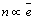(1)

We consider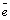as the estimate energy used for communication. The communication energyspent during one run of data forwarding from a source to sink is taken to be the linear combination of the product of probability of utilization and the energy spent in doing so. Since a node can act as both a source and relay,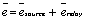. Substituting the probabilities: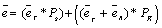(2)

Where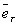and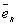the estimated energy used to transmit and receive the packets respectively and PS and PR are the probability of acting as a source and relay respectively. The energy estimates used here are corresponding to the energy spent during that action in one round of packet is forwarded from source to sink. We consider the scenario where we have a single sink and m sources where 0<m< total number of sensors T. Let the source nodes be S1, S2, S3 … Sm and let the probability of the source Si to act as the source in a query plan be pi. Thus, for a sensor j, the probability of acting as a relay is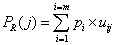(3)

Here uij is the probability of sensor j to act as a relay node for a transmission originating from the ith sensor as the source. uij is a probability estimate and its estimation especially in an irregular topology is dependent on various physical and deployment factors. The accuracy of the estimate dictates the efficacy of the proposal. The scheme does not lay any rule to calculate the probabilities but an experimental procedure of determining the probabilities by running a simulation of the experiment should be best suited. The values can also be estimated by using heuristics.

Substituting equation (2) and equation (3) into equation (1) we get the number of redundant nodes around jth sensor,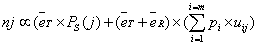(4)

Since, this equation only utilizes the energy spent during data forwarding it can be generalized even further by adding the term eothers, which would be estimate of energy spent during other tasks like sensing, aggregation etc.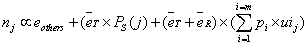(5)

To calculate actual numbers we need to decide upon a constant condition. We assume that the total number of redundant sensors available has an upper limit N. Hence,

N=Σnj

Also, the energy dissipated at each level should be normalized. Hence, for sensors Si and Sj:

nj/ni = ej/ei

The two equations are sufficient to solve for all n by assigning any one of the n values.

Now we try to elaborate the equation for two special cases where:

1) Each node acts as a source, forwarding data to the sink at equal intervals,

2) Only the end nodes or the nodes in the outermost rings act as the source

We try to use the number of sensors at a hop-distance to estimate the values for various variables declared above.

Let t1, t2, t3 … tn be the total number of sensors at 1, 2, 3 and n hop distances from the sink. The participation of a sensor at k-hop distance in a query as a relay node is only practical when it acts as a relay node for queries originating from sensors at hop-distances >k from the sink.

Scenario 1: Every active sensor acts as a source

Assume that the furthest node from the sink is at n-hop distance from the sink.

Here, PS = 1/Σti = 1/T, as the probability of a sensor acting as a source is 1/total number of sensors (T). Now, as each sensor acts as a source with equal probability.

From equation (3)Here,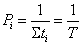(6)

Also, uij =0, if source sensor Si is at hop distance <k as the sensors at the kth hop distance would not be utilized to act as a relay node. The value of uij =1/tk if source sensor Si is at hop distance >k as the participation of a sensor Sk to act as a relay node is shared by all the tk sensors at k-hop distance from the sink.

For a sensor at k-hop distance from the sink, to calculate PR(j) we would require to summate over the sources at a hop-distance >k. The total number of such source nodes would be a summation of all nodes at hop-distance >k. Thus, the number of source nodes becomes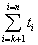.

Substituting pi in eq (3),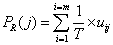(7)

Substituting the total number of effective source nodes in equation (7),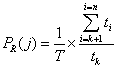(8)

Substituting equation (8) in equation (5),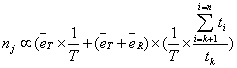(9)

Scenario 2: A sensor at n-hop distance from the sink acts as a source

Here, PS=0 for relay nodes, as only the sensors at n-hop distance from the sink act as source nodes.

From eq(3)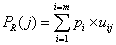Here,

pi=1/tn    (10)

Also, uij=1/tk      (11)

As the participation of a sensor Sk to act as a relay node is shared by all the tk sensors at k-hop distance from the sink.

For a sensor at k-hop distance from the sink, to calculate PR(j) we would require to summate over all the sources. The total number of source nodes would be tn as only the nodes at n-hop distance act as source nodes.

Substituting pi in equation (3),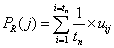(12)

Substituting uij in equation (12),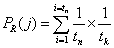(13)

Substituting equation (13) in equation (5),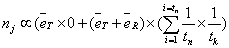(14)

In the next section, we compare the network lifetime obtained by using the proposed scheme using equation (4), equation (9) and equation (14) against two popular node distribution models i.e., an increasing node distribution for redundant nodes from source to sink and a uniform distribution of redundant nodes.

#### Experiment and Results

In this section, we compare the network lifetime of the proposed scheme against two popular node distribution models i.e., an increasing node distribution for redundant nodes from source to sink and a uniform distribution of redundant nodes. We compare the network lifetime of the sensor networks (Y-axis) over total budget of redundant sensors (X-axis) in the network simulated using simulation parameters defined in Section 9 over these six different scenarios:

I. A 4-neighbour, SSOP model where each node acts as a source with equal probability (results in Figure 6(a)),

Figure 6: Comparing network lifetime of sensor network between proposed schemes, uniform and geometrically increasing node density for 4-Neighbour SSOP topology when (a) Every active sensor acts as a source, (b) outermost nodes act as source nodes, (c) m sources each at different hop distance from the sink act as source.

II. A 3-neighbour, SSCP model where each node acts as a source with equal probability (results in Figure 7(a)),

Figure 7: Comparing network lifetime of sensor network between proposed schemes, uniform and geometrically increasing node density for 3-Neighbour SSCP topology when (a) Every active sensor acts as a source, (b) outermost nodes act as source nodes (c) m sources each at different hop distance from the sink act as source.

III. A 4-neighbour, SSOP model where only the outermost nodes act as source nodes (results in Figure 6(b)),

IV. A 3-neighbour, SSCP model where only the outermost nodes act as source nodes (results in Figure 7(b)),

V. A 4-neighbour, SSOP model where there are m source nodes, each at a different hop-distance from the sink and are transmitting data to the sink in unequal time intervals (results in Figure 6(c)),

VI. A 3-neighbour, SSCP model where there are m source nodes, each at a different hop-distance from the sink and are transmitting data to the sink in unequal time intervals (results in Figure 7(c))

In Figures 4(a) and 5(a) we have seen a case where when each sensor node acts as source node, the increasing node distribution for redundant nodes out-performs the uniform distribution and contrastingly through Figures 4(b) and 5(b) (Section 5), the uniform distribution out-performs the former when only the outermost sensors act as source nodes. We also observed how the SSCP model slightly favours the increasing distribution model and the SSOP model favours the uniform distribution model. In Figures 6(a) and 7(a) we extended our experiment to compare these results against the numbers obtained from the proposed scheme. In Figures 6(a) and 7(a) we can see how the proposed scheme out-performs both the distribution schemes for the SSOP model and nearly matches the numbers for increasing distribution for the SSCP model. Also, through Figures 6(b) and 7(b) we can observe a similar trend where the proposed model outperforms both the distribution schemes for an SSCP model and nearly matches the numbers obtained for uniform distribution for the SSOP model. Thus, as proposed, the scheme is able to generalize the trends mentioned above. We obtained the numbers for a generalized scenario where m different sensors act as source nodes forwarding data at unequal intervals for both SSOP and SSCP models. This is done to observe the three distribution schemes performance for a generalized model and in both cases; the proposed scheme is able to out-perform the other distribution schemes.

#### Simulation Environment

For the purpose of simulation, we have used AlgoSenSim to simulate the WSN environment for various cases. AlgoSenSim performs the simulation in iterations called generations. Each generation is basically an instance in time in which it evaluates all the tasks that have to be performed across all nodes in the network. A task it performs at each node is called an Algorithm. We used the Power-DSAP (Directional Source-Aware Protocol)  scheme, a power efficient greedy routing algorithm, as the routing algorithm for the purpose of simulation. Power-DSAP has the advantage of being able to be used as a powerefficient routing scheme and the flexibility of using it with 3-neighbour, 4-neighbour generalized to n-neighbour mesh topologies. The metrics on which the evaluation is done is network lifetime. So, for the purpose of the tests, the number of generations in AlgoSenSim it takes for the first cluster to fail is taken as the estimate of network lifetime.

Now, we give a brief description of assumptions made for simulation. Each packet will be of fixed size i.e., 1000 bytes, originating from a source to a single static sink. For battery usage, we assume that only the energy spent in message transmission in the network, contribute to depletion in energy. We also assume that at any point of time only 1 sensor is active in the cluster and all other nodes (acting as redundant sensors) are in a Sleep State.

For each simulation, the total number of nodes in the budget was varied from 100 to 1000 and the results are plotted to see the effect of number of total nodes on the experiment.

#### Conclusion

In this paper we contemplated about the energy-hole problem persisting in wireless sensor network and how placing redundant nodes around sensors receiving more traffic can substantially increase the lifetime of the sensor network. We first discussed that how the previous studies were able to resolve the same for a model where each sensor can act as a sink and how increasing network density from source towards sink can solve that problem. In general, abiding by the concept of application specific network design, we assume, given that initial position of nodes are decided based on application parameters like terrain, areas to monitor, region accessibility etc., there should be a way to decide that in a budget of total N sensors, how can we distribute the redundant nodes around each sensor to increase the network lifetime. We showed that a single model for density distribution is not favourable for multiple cases. Thus, we generalize the trends using a simple equation. We see how it is effective in resolving the problem for different cases and can be generalized using experimentally or heuristically computed estimations. The future work is to generalize for irregular topologies and a larger variance of routing schemes. This enables us to decide how effectively we can distribute the power consumption among the limited resource in hand, in turn increasing network utilization and lifetime.

#### References

Select your language of interest to view the total content in your interested language

### Article Usage

• Total views: 13970
• [From(publication date):
December-2015 - Dec 08, 2019]
• Breakdown by view type
• HTML page views : 9955Can't read the image? click here to refresh